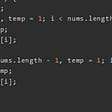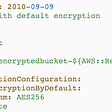# Ideas and Solutions for Advent of Code 2021 in Kotlin — Part 3/4

The third week of Advent of Code requires more time and even some competitive programming knowledge like dynamic programming and graph theory. In this article, I share some high-level ideas and my solutions if you need a hint or a few to get that gold star.

Ideas and Solutions for tasks 1 to 6 can be found here, for tasks 7 to 12 — here.

## Day 13: Transparent Origami

The 2D array contains two types of symbols — `.` and `#`. This array can be folded horizontally and vertically multiple times. When folding `#` symbols replace `.` symbols, but not vice versa. Here is the complete task.

This is a modeling task, which you can do on the 2D array itself, but the possible range is quite large, so you can hit a memory limit. The smarter solution would be to fold the `#` symbols (the initial input, actually).

Such folding can be done with Kotlin’s `fold` function using the initial value of `#` symbol positions and `mapNotNull`, which mirrors X or Y coordinates depending on the fold direction.

Here is my solution.

## Day 14: Extended Polymerization

We get the initial string (template) consisting of uppercase letters, which describe the initial state of the polymer. The list of pair insertion rules allows growing the initial polymer step by step exponentially. We need to model this growth during 10 (first sub-task) and 40 (second sub-task) steps. Here is the complete task.

The first sub-task can be solved just by modeling, but even 20 steps are too long and require too much memory and time. The (much) better solution is based on dynamic programming.

You can easily see that every pair grows independently of others (new elements are always added inside the pair). It means that the solution can be found for each pair separately and then combined. It doesn’t speed up things sufficiently though. But makes the solution easier? Yes.

Now, let’s grow each pair step by step. So at each step, every pair (usually) produces two more pairs, which have one less step to produce pairs in their turn. If we start from step zero (template), we know which pairs we have, so the value for them is one. Then on step one, we combine values for produced pairs from step zero, and so on.

The only complication here is that for each step and pair of symbols we need to store the complete array of symbols counts from `A` to `Z`, which requires a 3D array as a result — yeah, 3D dynamic programming ;)

Here is my solution.

## Day 15: Chiton

Another 2D array to find the shortest path this time between the start point and the endpoint. There is a complication in the second sub-task, which requires us to unfold the initial array 5 times, which makes it 25 times larger. Here is the complete task.

Due to the limited range of values in the array (from 1 to 9), moving only to the right and bottom works on the first sub-task, but not on the second. The expected solution is an optimized Dijkstra’s algorithm (with asymptotic of NlogN) or Bellman-Ford algorithm.

Here is my solution.

## Day 16: Packet Decoder

We are provided with a hexadecimal string, which represents a hierarchy of packets. Packets can either be literals or operations executed on literals or results of other operators. Operators can include 0, 1, or multiple other operators and/or literals. Here is the complete task.

The first sub-task is about parsing a hexadecimal string to the hierarchy of packets and summing up a part meta-data (version). There are a few tips, which can help you implement the parsing in a simple and effective way:

• `Char.digitToInt(radix:)` helps you to convert a single hexadecimal symbol to decimal `Integer`, then `toString(radix:)` and `padStart(length:, padChar:)` makes it easy to get binary chunks of 4 bits.
• The resulting binary string can be parsed packet by packet taking bits from the left while the current packet requires some input, then reassigning what’s left, and continuing with the next packet.
• There are 3 distinct cases to parse: literal, operator with a length, and operator with a count. Each case can be implemented as a separate function that returns parsed packet and string that’s left unparsed. `String.substring(range:)` will be very handy here.

Once you have the hierarchy of packets (have you used `sealed classes` to represent `Literal` and `Operator` cases?), calculating the actual value of the top-most operator is very straightforward. All you need is a single-line operation on sub-packets using `map`, `minOf`, `maxOf`, `sumOf`, and other well-known collection functions per each operator type.

Here is my solution.

## Day 17: Trick Shot

In this task, we make shots in 2D space with different initial X and Y velocities to hit the target — rectangular area. Velocities are changed with each modeling step — X-velocity is decreased by drag, Y-velocity is decreased by gravity. Here is the complete task.

In nutshell, we need to brute force all possible start velocities (I used ranges of `0..1000` for X-velocity and `-1000.1000` for Y-velocity), and then model the shot to see if it hits the target.

In the first sub-task, we need to find the shot, which reaches the maximum Y-coordinate but still hits the target. In the second sub-task, we need to simply count all shots that hit the target.

Here is my solution.

## Day 18: Snailfish

We are provided with a list of binary trees, which represent a pair-number. Pair-number is an ordered list of two elements. Each element of the pair can be either a regular number or another pair. To add two pair-numbers, we form a pair from the left and right parameters of the addition operator.

There are two operations that we need to implement on these binary trees when certain conditions arise — explode and split. The “explode” operation replaces any pair of regular numbers with a depth of 5 by a 0 and adds the left number of the nearest number on the left, the right number — to the nearest number on the right. The “split” operation creates a pair-number of regular numbers from a number value of which exceeds 9. Here is the complete task.

Both subtasks require implementing those two operations (explode and split) and calculating the magnitude of the resulting pair-number from some subset of input data. To me the biggest challenge was that the “explode” operation can be implemented much easier on the string representation, while the “split” and “magnitude” are much easier on the real binary tree.

I’ve ended up working with both representations at the same time by converting them to binary trees from string and vice versa on every iteration. I’m sure it can be done in a more straightforward way, so, please, link your solution in the comments.

From the Kotlin perspective, I’ve found sealed classes very useful to represent the binary tree (Node -> Pair, Number). `substringBefore`, `substringAfter`, `substring`, `slice`, `indexOfFirst`, `indexOfLast` are just some functions, which I’ve used in this task to simplify my solution.

# Bottom line

Those were the third 6 tasks for the Advent of Code. I hope that the ideas and solutions shared here will help you to get another perspective on solving algorithmic tasks with Kotlin.

If your solutions are based on different ideas or are written in different languages, or you simply want to share your solution as well, don’t hesitate to comment on this article. And good luck for the rest of the advent!

--

--

--

## More from xorum.io

Kotlin Multiplatform solutions for Mobile (iOS | Android) and Web (Ktor | React.js)

## SpiderDAO deploys Bugzilla!## Compiling ‘C’ files with ‘gcc’## Online Freelancing (Part 5): How to manage Clients?## Azure Monitor Log Analytics too Expensive? Part 2 — Cost Optimization## Easier AWS CloudFormation## NETWORK DOCUMENTATION 101## Yev Kanivets

Professional Mobile Engineer since 2014. Kotlin Multiplatform enthusiast. Love hackathons, competitive programming, and challenging tasks. Marathon finisher.

## Ideas and Solutions for Advent of Code 2021 in Kotlin — Part 4/4## Kotlin Multiplatform library with Kotest and Gradle Version Catalog## Kotlin ⚡️: Null Safety | Erselan Khan## The magic of RxJava with a bit of Kotlin awesome collections tools